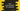# How to get the ASCII value of a character in dart# Introduction :

In dart, strings are a sequence of UTF-16 code units. Or each character is represented as UTF-16. Dart String class provides two different methods to get the code unit of a character in the string or to get all code units.

In this tutorial, we will learn how to print the code unit of each character in a string one by one and how to get all code units of all characters.

Following are the methods that we are going to use :

## String.codeUnitAt :

This method is used to print the code unit of a character in the string using its index value. The index starts from 0 i.e. 0 is the index of the first character, 1 is the index of the second character, etc.

This method is defined as below :

``int codeUnitAt(int index)``

As you can see, it takes the index of the character as the parameter and returns one int value, i.e. the code unit for that character.

## String.codeUnits :

This method is used to get all code units of string characters. It is defined as below :

``List codeUnits``

It returns a list with all code units of the string characters. The list is unmodifiable.

### Example to print all code units of a string :

Let’s learn the above methods with an example. We will print the code units of each character of a string one by one using codeUnitAt, and also we will print the list of all code units in the string :

``````void main() {
String s = "Hello World";

for (int i = 0; i < s.length; i++) {
print("Code unit for \${s[i]} is \${s.codeUnitAt(i)}");
}

print("Code unit list \${s.codeUnits}");
}``````

### Output :

``````Code unit for H is 72
Code unit for e is 101
Code unit for l is 108
Code unit for l is 108
Code unit for o is 111
Code unit for   is 32
Code unit for W is 87
Code unit for o is 111
Code unit for r is 114
Code unit for l is 108
Code unit for d is 100
Code unit list [72, 101, 108, 108, 111, 32, 87, 111, 114, 108, 100]``````